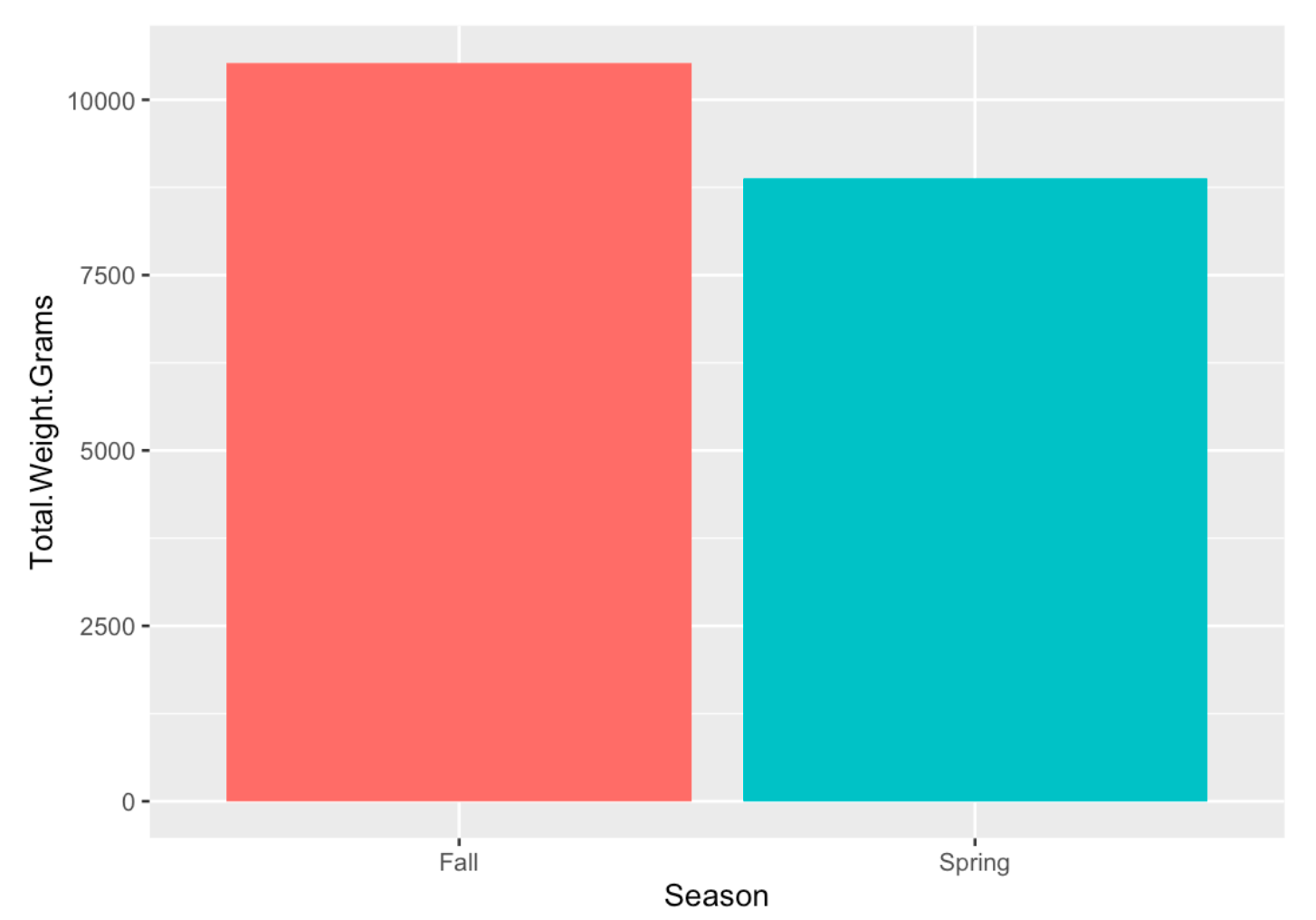Late in May, I collected and analyzed data on the one-year-old clams before they began feeding and rapidly growing. I was specifically focused on the average clam shell length and the estimated total number of live clams we had in the upweller. Our goal was to compare this data to the data that we collected on the same clams in the Fall of 2021. We wanted to determine what percentage of clams survived the winter in the overwintering tank, as well as if the clams grew at all over the winter.

All of our clams were split into nine mesh-bottom buckets. In order to calculate the estimated number of clams per bucket, I took five samples of clams from each bucket. With each sample, I counted all of the live clams and then calculated the sample weight using a scale and petri dish (the samples included live clams and empty shells). Using the sample clam count and sample clam weight, I was able to calculate how many clams were in one gram. Once all five samples were collected for all nine buckets, I collected data on the total weight (including empty shells) of the contents in each bucket. To do this, I transferred the clam contents in each bucket, removing as much water weight as possible, into a tared, dry bin. Using the total weight of the contents in each bucket as well as the estimated number of clams per gram, I was able to calculate five estimates for the number of clams in each bucket. I averaged out the five sample estimates to get one estimated number of clams in each bucket. Finally, I calculated the estimated total number of clams by finding the sum of the estimated number of clams in each bucket. We estimated that we had a total of 27,465 clams in the upweller. In the fall, we estimated that we had about 33,719 total clams. Therefore, roughly 81.45% of the clams survived the winter. This survival rate is much higher than survival rates in the wild where they must deal with predators such as green crabs and ribbon worms.Figure 1: A bar graph comparing the total weight of the clam contents in the Fall of 2021 with the Spring of 2022.

The clam mortality we experienced could be caused by many factors. One possible factor could be due to packing the clams too tightly in the overwintering bags. Clams start feeding when the water temperature is warm enough. If the water temperature triggered the clams to start feeding while they were still packed in the overwintering bags, they wouldn’t have enough room to stick their siphons out. It would be helpful to know the exact water temperature the clams start feeding at so we can quickly transfer them to buckets where they would be more spread out.Figure 2: Comparing the average shell length of the clams in the Fall of 2021 with the Spring of 2022. The dot represents the mean clam shell length and the upper and lower bars represent the 95% confidence interval.

Additionally, using a caliper, I measured the shell lengths of a sample of  35 clams from each bucket. Once this data was collected, I calculated the average shell length along with a 95% confidence interval for each bucket. Using these calculations, I determined the mean clam shell length and a 95% confidence interval among all nine clam buckets. The mean clam shell length for all clams was 13.3341 mm and we are 95% confident that the true mean clam shell length is between 12.48659 mm and 14.1816 mm. In the fall, we calculated a mean clam shell length of 12.83716 and we were 95% confident that the true mean clam shell length was between 11.93104 mm and 13.74328 mm. In conclusion, our clams experienced almost no growth over the winter – an average of only 0.49694 mm. These results were expected because as mentioned earlier, soft-shell clams go dormant throughout the winter until the water temperature increases enough to trigger the clams to begin feeding and growing. This data has helped us answer some important questions allowing us to be better prepared for the next batch of clams that we will be overwintering.